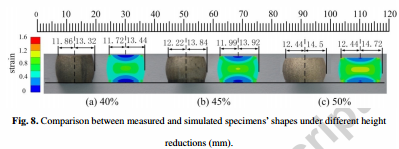# Identification of friction coefficients and strain-compensated Arrhenius-type constitutive model by a two-stage inverse analysis technique

Author(s):
Zhang Cunsheng, Ding Jie, Dong Yuanyuan, Zhao Guoqun, Gao Anjiang, Wang Lanjun (Shandong University)

CHALLENGE - A reasonable constitutive model plays an important role in the accurate simulation of aluminum alloy extrusion process. In this work, a two-stage inverse analysis technique is proposed to identify the friction coefficients during hot compression test of aluminum alloy 6N01 (AA6N01) and its material parameters in the strain-compensated Arrhenius-type constitutive model.

SOLUTION -  Firstly, the minimal shape error between the measured and simulated specimen is set as the optimization objective. The friction coefficient under each forming condition is obtained by the first-stage inverse analysis method. Secondly, 16 unified material parameters in the constitutive model are identified by the second-stage inverse analysis method. The two stages are combined together to realize loop calculation: the first-stage inverse analysis provides friction coefficients for the identification of material parameters while the second-stage updates new material parameters for identifying better friction coefficients. When the average error (Er(friction)) between friction coefficients obtained at the current and last loop is less than 5%, the whole identification process comes to the end and the optimized friction coefficients and material parameters are obtained.BENEFITS - Results show that the maximum shape error is only 2.48%, which indicates that the obtained friction coefficients can reflect the practical lubrication conditions between the tooling head and specimens. The global predicted error is only 4.2%, which verifies that the proposed model and obtained material parameters can describe well the rheological behavior of AA6N01 at elevated temperatures. By means of the optimization software modeFRONTIER, FEM simulation and error calculation are integrated in this paper, identifying friction coefficients and constitutive parameters. The SIMPLEX algorithm is adopted in this work due to its good characteristics of simple calculation, fast convergence speed and high accuracy.